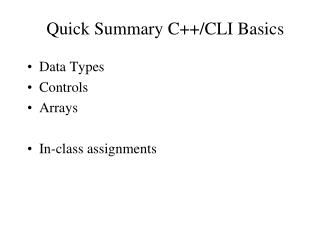Download PresentationQuick Summary C++/CLI Basics

# Quick Summary C++/CLI Basics

Download Presentation## Quick Summary C++/CLI Basics

- - - - - - - - - - - - - - - - - - - - - - - - - - - E N D - - - - - - - - - - - - - - - - - - - - - - - - - - -
##### Presentation Transcript

1. Quick Summary C++/CLI Basics • Data Types • Controls • Arrays • In-class assignments

2. Namespaces namespaceMyNamespace { // …. } MyNamespace::func1() using namespace OtherNamespace; Comments: // /* xxxx */

3. Basic Data Types • Type • Range • Literals/constants and variables • Operators and expressions • Assignment • Input and output

4. Primitive Data Types in C++/CLI • Integer: • Int16 (short); UInt16 (unsinged short) • Int32 (int or long); UInt32(unsigned int or long) • Int64 (long long); UInt64(unsigned long long, _int64) int x, y(1), z = 123; (signed, 32 bits) int x = y = z = 200; Console::WriteLine(0x10); Console::WriteLine(-0x10); // negative hex 10 is base-10 -16 • Floating: • Single (float, 32bits) • Double (double, 64bits)

5. Primitive Data Types in C++/CLI • Boolean (bool, true or false) • Character (Char, 16bits, unsigned unicode) • Character and escape sequence chara = ‘A’; // character ‘A’ Charb = L‘A’; // unicode ‘A’ Char c = L‘\x0041’; // hex 41 is ASCII ‘A’ chard = ‘\t’; // tab escape Char e = L‘\\’; // unicode backslash escape

6. Handles vs Pointers Handle: safe code Pointer: unsafe code Pointer: * Reference data in C Run-Time heap, need to handle memory management yourself pointer’s address can be manipulated Return from new after creating an instance of a reference type object in CRT heap • Handle: ^ • Reference data in managed heap (CLR offers memory management) • Handle’s address can not be manipulated (no add or subtract offsets to it • Return from gcnew after creating an instance of a reference type object in managed heap

7. Operators, Expressions and Assignments, Precedence and Associativity • Arithmetic Operators • Unary Operators • Bitwise and Shift Operators • Relational Operators • Logical Operators • Ternary Operator

8. Quick Summary C++/CLI Basics • Data Types • Controls • Arrays • In-class assignments

9. Control Structures • Structured Programming • Selection • if • if else • switch(break) • Repetition (break, continue) • while • do … while • for

10. break & continue int sum=0; for (int i=1; i<=10; i++) { if (i%5==0) continue; sum += i; // sum = sum+i; } int sum=0; for (int i=1; i<=10; i++) { if (i%5==0) break; sum += i; // sum = sum+i; }

11. Quick Summary C++/CLI Basics • Data Types • Controls • Arrays • In-class assignments

12. Arrays • Arrays • One dimensional array • Two or multiple dimensional array

13. Array in C++/CLI • Array, element, index(or subscript) • array<int> ^ a = gcnew array<int>(12); • array<int> ^ a = {31, 28, 31, 30, 31, 30, 31, 31, 30, 31, 30, 31}; • a, a,…, and a • a->Length • array<String ^> ^d = {“Sun”, “Mon”, “Tue”, “Wed”, “Thu”, “Fri”, “Sat”}; • int main(array<System::String ^> ^args) {}

14. Array in C++/CLI • Multi-dimensional Array • array<int, 2> ^ b = gcnew array<int, 2>(4,3); • array<int, 2> ^ b = {{1, 2, 3}, {4, 5, 6}, {7, 8, 9}, {0, 1, 2}}; • b[0,0], b[0,1],…, b[3,2]; • b->Rank, b->GetLength(0), b->GetLength(1)

15. Quick Summary C++ for .NET • Data Types • Controls • Arrays • In-class assignments • Control • Array